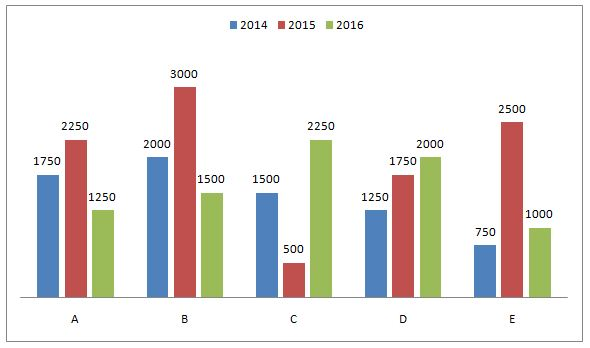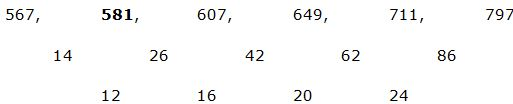# NIACL AO Prelims – Quantitative Aptitude Questions Day- 71

Dear Readers, Bank Exam Race for the Year 2019 is already started, To enrich your preparation here we have providing new series of Practice Questions on Quantitative Aptitude – Section. Candidates those who are preparing for NIACL AO Prelims 2019 Exams can practice these questions daily and make your preparation effective.

[WpProQuiz 4807]

Directions (1 – 5): Find the wrong term in the following number series

1) 172, 200, 263, 388, 604, 947, 1459

a) 263

b) 388

c) 200

d) 604

e) 947

2) 9, 16, 45, 175, 875, 5244

a) 175

b) 45

c) 875

d) 5244

e) 16

3) 34, 32, 62, 184, 732, 3668

a) 32

b) 3668

c) 184

d) 732

e) 62

4) 567, 579, 607, 649, 711, 797

a) 607

b) 579

c) 711

d) 797

e) 649

5) 91, 92, 187, 565, 2271, 11364

a) 2271

b) 92

c) 11364

d) 187

e) 565

Directions (6 – 10): Study the following information carefully and answer the given questions:

The following bar graph shows the total number of items produced by five different companies in three different years.6) Number of items produced by company A, C and E together in 2014 is approximately what percentage of the number of items produced by company B, C and D together in 2015?

a) 70 %

b) 76 %

c) 55 %

d) 90 %

e) 65 %

7) Find the total number of items produced by company A and B together in all the given years together?

a) 11750

b) 12500

c) 12250

d) 12625

e) None of these

8) Number of items sold by all the given companies in 2014 is approximately what percentage more than the number of items sold by company C in all the years together?

a) 40 %

b) 80 %

c) 71 %

d) 50 %

e) 60 %

9) Find the difference between the total number of items sold by all the companies together in 2015 to that in 2016?

a) 2000

b) 5000

c) 3000

d) 4000

e) None of these

10) Find the respective ratio between the total number of items sold by the company B and E in 2014 to that of the total number of items sold by A and D in 2016?

a) 12: 23

b) 13: 15

c) 19: 12

d) 11: 13

e) None of these

Directions (1-5):

The correct series is,

172, 199, 263, 388, 604, 947, 1459

The pattern is, +33, +43, +53, +63, +73, +83,..

The wrong term is, 200

The correct series is,

9, 16, 45, 176, 875, 5244

The pattern is, *2 – 2, *3 – 3, *4 – 4, *5 – 5, *6 – 6,..

The wrong term is, 175

The correct series is,

34, 32, 62, 184, 734, 3668

The pattern is, *1 – 2, *2 – 2, *3 – 2, *4 – 2, *5 – 2,..

The wrong term is, 732

The correct series is,The difference of difference is, 12, 16, 20, 24…

The wrong term is, 579

The correct series is,

91, 92, 187, 566, 2271, 11364

The pattern is, *1 + 1, *2 + 3, *3 + 5, *4 + 7, *5 + 9,..

The wrong term is, 565

Directions (6-10):

Number of items produced by company A, C and E together in 2014

= > 1750 + 1500 + 750 = 4000

Number of items produced by company B, C and D together in 2015

= > 3000 + 500 + 1750 = 5250

Required percentage = (4000/5250)*100 = 76%

The total number of items produced by company A and B together in all the given years together

= > (1750 + 2250 + 1250 + 2000 + 3000 + 1500)

= > 11750

Number of items sold by all the companies in 2014

= > 1750 + 2000 + 1500 + 1250 + 750 = 7250

Number of items sold by company C in all the years together

= > 1500 + 500 + 2250 = 4250

Required percentage = [(7250 – 4250)/4250]*100 = 70.58% = 71 %

Total number of items sold by all the companies in 2015

= > 2250 + 3000 + 500 + 1750 + 2500 = 10000

Total number of items sold by all the companies in 2016

= > 1250 + 1500 + 2250 + 2000 + 1000 = 8000

Required difference = 10000 – 8000 = 2000

Total number of items sold by companies B, E in 2014

= 2000 + 750 = 2750

Total number of items sold by companies A and D in 2016

= 1250 + 2000 = 3250

Required ratio = 2750: 3250 = 11: 13